hashkat.org

# A Diverse Network of Agents

#k@ offers the opportunity for users to create network simulations consisting of a varied set of agents in a diverse world. The simulated population may be multilingual, multiregional and consisting of agents of different ideologies, follow, tweet and retweet preferences. While every model is simplified, #k@ strives to model the complexity and diversity of a social network in the real world.

In this tutorial we will show you how to create different regions and agent_types to give you the freedom to create the most realistic network simulation possible. This tutorial should take about 40 minutes to complete.

## What Makes an Agent Unique?

#### Ideology

An agent's ideology is coded into the signal to represent content. To the user, they may represent religion, political affiliation, or any social attitude where other agents may agree or disagree, and chose to follow on that basis.

Ideologies are specified in the 'ideologies' section of the configuration file INFILE.yaml. The default ideologies are listed below.

``````ideologies:
- name: Red
- name: Blue
- name: Green
- name: Orange
``````

The proportion of agents with a particular ideology in a region is configured via 'ideology_weights' in the 'regions' section of the input file.

``````regions:
- name: Ontario

ideology_weights: {Red: 100, Blue: 100, Green: 100, Orange: 100}
``````

The ideology weights are normalized, so the proportion of Red agents in Ontario will be 100/400 where 400 is the sum of the ideology_weights. ' Agents may follow other agents based on ideology under the 'hashtag' follow_model (or combination/'twitter' follow_model). The 'hashtag' follow_model must be enabled and 'care_about_ideology' under the agent_type set to 'true'. This permits agents to follow agents of the same ideology.

``````agents:
- name: Standard
hashtag_follow_options:
care_about_ideology: false # does the agent care about the ideology of the agent they will follow?
``````

#### Language

Agents in #k@ must speak at least one language, which may be English, French and English, French, or Spanish. The proportion of agents who speak a particular language is set in the 'regions' section of the configuration file under 'language_weights'.

``````regions:
- name: Ontario

language_weights: {English: 100, French: 0, Spanish: 0, French+English: 0}
``````

#### Region

A region is the geographical location of an agent. Regions may be named and configured in the 'regions' section of INFILE.yaml. The region's add_weight determines the proportion of agents in a simulation being from that region. In #k@ the add_weights of all regions are normalized, so Ontario's add weight would be 5 divided by the sum of the add_weights of all regions. If Ontario is the only region with a non zero add_weight, 100% of agents will be Ontarian, regardless of the actual number entered.

The proportion of the region's population with a certain ideology, language, and preference class are set as shown.

``````regions:
- name: Ontario

preference_class_weights: {NoRetweetPref: 100}
ideology_weights: {Red: 100, Blue: 100, Green: 100, Orange: 100}
language_weights: {English: 100, French: 0, Spanish: 0, French+English: 0}
``````

#### Preference Class

The preference class determines the probability that the tweets of an agent will be retweeted. Retweeting is called tweet_transmission. Retweet preference classes may be configured for both tweet_type and agent_type.

In this section of the program we may use the word else to include all unnamed agent_types or all to include all agent_types without naming them.

``````preference_classes:
- name: StandardPref

tweet_transmission:
plain: # Also applies to musical tweets
Standard: 0.01
Celebrity: 0.01
else: 0.0
different_ideology: # generally no retweeting of tweets with different ideological content
all: 0.0
same_ideology:
Standard: 0.01
else: 0.2
humorous:
Standard: 0.02
Celebrity: 0.02
else: 0.4
``````

Preference_class_weights for the population in a region are set as follows.

``````regions:
- name: Ontario

preference_class_weights: {NoRetweetPref: 100}
``````

## Agent Configuration

Agents, the nodes of the graph, are a complex component of #k@ and require a number of configuration settings. Agents are defined in the 'agents' section of INFILE.yaml.

Add_weight is the proportion with which agents of a given agent_type are added to the network.

``````agents:
- name: Standard
weights:
# Weight with which this agent is created
``````

In #k@ agent add weights are normalized. If there are two agent_types, one of add weight 120 and the other of add weight 80, then the first type of agent will be 60% of agents in the network (120/200) and the second 40% (80/200).

#### Agent - Weights: follow

Follow weight is the proportion with which agents of a particular agent_type are followed compared to other agent types. Follow weight is used in the agent and preferential_agent follow_models.

``````agents:
- name: Standard
weights:
# Weight with which this agent is followed in agent follow
follow: 100.0
``````

Follow weights are also normalized in #k@.

#### Agent - Tweet Type

Tweet_type the frequency with which agents of a particular agent_type make tweets. The tweet_types are 'plain', 'ideological', 'humorous', and 'musical'. In the example below, each tweet_type has a value of 1.0. These values are normalized, so for every tweet the agent makes, there is a 1/4 chance it will be 'plain', 'ideological', 'humorous', or 'musical'.

``````agents:
- name: Standard
weights:
tweet_type:
ideological: 1.0
plain: 1.0
musical: 1.0
humorous: 1.0
``````

#### Agent - Followback Probability

Followback_probability is the probability that if an agent is followed, the agent will return the favour. On Twitter experiments have shown this value to be about 44%.

``````agents:
- name: Standard
# Probability that following this agent results in a follow-back
followback_probability: .44
``````

In order to use followback_probability use_followback must be set to 'true' in the 'analysis' section of INFILE.yaml.

``````analysis:
use_followback:
true
``````

#### Agent - Hashtag Follow Options

If the hashtag follow_model is chosen, agents may follow another agent based on hashtag in a tweet. Hashtags enable users to connect to a section of the network they may not previously have had a connection with.

To use the hashtag follow_model or combination ('twitter') follow_model, use_hashtag_probability's value must not be zero. Use_hashtag_probability is a probability from 0 to 1 that a tweet in the system will contain a hashtag.

In the agent settings, hashtag_follow_options determine which hashtags a particular agent_type will follow. Specifically, in #k@ agents may care to follow agents with hashtag tweets indicating they come from a particular region, or have a certain ideology.

These settings are called care_about_region and care_about_ideology and one or both should be set to 'true' for an agent to follow based on hashtags.

``````agents:
- name: Standard
hashtag_follow_options:
care_about_ideology: true # does the agent care about the ideology of the agent they will follow?
``````

Follow rate dictates the number of follows an agent of this agent_type will make per simulated minute.

Follow rate is a function which may be either 'constant' or 'linear'. For a constant follow rate, the function must be set to 'constant' and it must have a 'value' of 0 or greater assigned to it (a zero follow rate will result in no follows).

``````agents:
- name: Standard
rates:
# Rate for follows from this agent:
follow: {function: constant, value: 0.0001}
``````

For a linear follow rate, the function must be set to 'linear' and a 'slope' and 'y_intercept' specified. The 'y_intercept' is the initial rate of follows per simulated minute (like 'value' above) and the 'slope' is the amount the rate increases per simulated month.

``````agents:
- name: Standard
rates:
# Rate for follows from this agent:
follow: {function: linear, y_intercept: 0.1, slope: 0.1}
``````

Note: the add rate may also be a linear function.

#### Agent - Tweet Rate

The tweet rate sets the number of tweets an agent_type makes per simulated minute. As with follow rate, a function of this rate may be 'constant' or 'linear'. Below is an example of a constant function.

``````agents:
- name: Standard
rates:
# Rate for tweets from this agent:
tweet: {function: constant, value: 0.0001}
``````

This is an example of a linear tweet rate function.

``````agents:
- name: Standard
rates:
# Rate for tweets from this agent:
tweet: {function: linear, y_intercept: 0.1, slope: 0.1}
``````

To increase the number of ideologies, regions, preference_classes or agent_types in the simulation, change the value for N_BIN_IDEOLOGIES, N_BIN_REGIONS, N_BIN_PREFERENCE_CLASS, and/or N_BIN_AGENT_TYPES in the ../hashkat/src/config_static.h file.

Note: If you change ../src/ files make sure to then rebuild #k@ by running build.sh -O for the changes to take effect.

## A Complex Simulation

Now that we have seen the agent settings, we will create our most complex network simulation yet. For this network simulation, we would like to have the following:

• constant number of 100 agents
• maximum simulation time of 1000 simulated minutes
• maximum real time 10 minutes
• 'twitter' (combination) follow_model implemented, with 'twitter_suggest' (preferential_global) and the 'hashtag' follow_models evenly weighted and other follow_models set to zero
• stage1_unfollow: false
• unfollow_tweet_rate: 0.0
• use_hashtag_probability: 0.5
• use_followback: true
• use_follow_via_retweets: true
• the 4 default ideologies will be used: Red, Blue, Green, Orange
• two regions will be configured, 'Ontario' and 'Quebec. Ontario will have an add rate of 3, Quebec add rate of 2 and a 'Mexico' add rate of 0
• Ontarians will have preference_class_weights: {StandardPref: 1}, ideology_weights: {Red: 25, Blue: 25, Green: 25, Orange: 25} and language_weights: {English: 50, French: 50}
• Quebecers will have preference_class_weights: {NoRetweetPref: 1}, ideology_weights: {Red: 25, Blue: 25, Green: 25} and language_weights: {English: 50, French: 50}
• 'StandardPref' preference class, set 'tweet_transmission' rates for the agent types to 0.1 for 'plain' & 'musical' tweets, 0.0 for 'different_ideology' tweets, 0.2 for 'same_ideology' tweets, and 0.4 for 'humorous' tweets
• 'NoRetweetPref' preference class will remain the same, with a value of 0 for all variables

We would also like you to use only the following 5 agent_types with their respective characteristics. Place the code for the new agent_types in INFILE.yaml. The code may also be found at /hashkat/docs/tutorial_input_files/tutorial12/.

#### Standard - Self-Centered Chatty Agent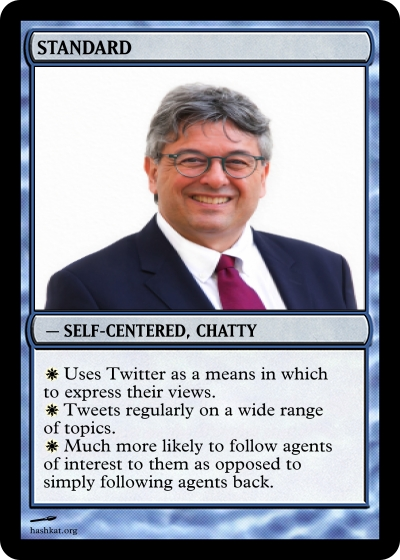``````- name: Standard-Chatty
weights:
# Weight with which this agent is created
# Weight with which this agent is followed in agent follow
follow: 5.0
tweet_type:
ideological: 1.0
plain: 1.0
musical: 1.0
humorous: 1.0
# Probability that following this agent results in a follow-back
followback_probability: 0.2
hashtag_follow_options:
care_about_ideology: true # does the agent care about the ideology of the agent they will follow?
rates:
# Rate for follows from this agent:
follow: {function: constant, value: 0.05}
# Rate for tweets from this agent:
tweet: {function: constant, value: 0.5}
``````

#### Standard - Friendly Networking Agent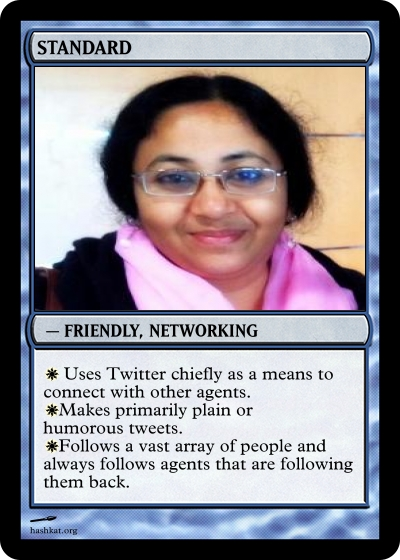``````- name: Standard-Friendly
weights:
# Weight with which this agent is created
# Weight with which this agent is followed in agent follow
follow: 5.0
tweet_type:
ideological: 1.0
plain: 7.0
musical: 1.0
humorous: 3.0
# Probability that following this agent results in a follow-back
followback_probability: 1.0
hashtag_follow_options:
care_about_ideology: false # does the agent care about the ideology of the agent they will follow?
rates:
# Rate for follows from this agent:
follow: {function: constant, value: 0.3}
# Rate for tweets from this agent:
tweet: {function: constant, value: 0.04}
``````

#### Standard - Funny Social Commentator Agent``````- name: Standard-Funny
weights:
# Weight with which this agent is created
# Weight with which this agent is followed in agent follow
follow: 5.0
tweet_type:
ideological: 1.0
plain: 1.0
musical: 0.0
humorous: 6.0
# Probability that following this agent results in a follow-back
followback_probability: 0.44
hashtag_follow_options:
care_about_ideology: false # does the agent care about the ideology of the agent they will follow?
rates:
# Rate for follows from this agent:
follow: {function: constant, value: 0.02}
# Rate for tweets from this agent:
tweet: {function: constant, value: 0.1}
``````

#### Standard - Music Advocate Agent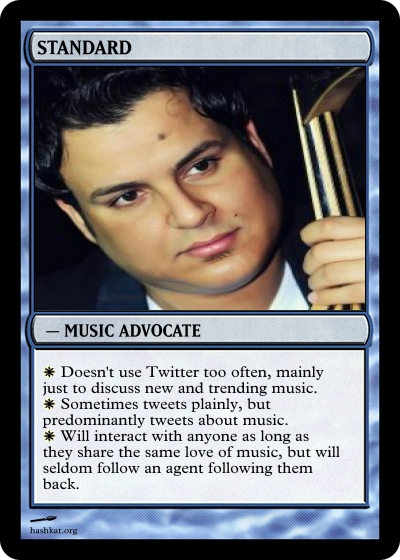``````- name: Standard-Musical
weights:
# Weight with which this agent is created
# Weight with which this agent is followed in agent follow
follow: 5.0
tweet_type:
ideological: 0.0
plain: 1.0
musical: 5.0
humorous: 0.0
# Probability that following this agent results in a follow-back
followback_probability: 0.1
hashtag_follow_options:
care_about_ideology: false # does the agent care about the ideology of the agent they will follow?
rates:
# Rate for follows from this agent:
follow: {function: constant, value: 0.01}
# Rate for tweets from this agent:
tweet: {function: constant, value: 0.02}
``````

#### Standard - Political Satirist Agent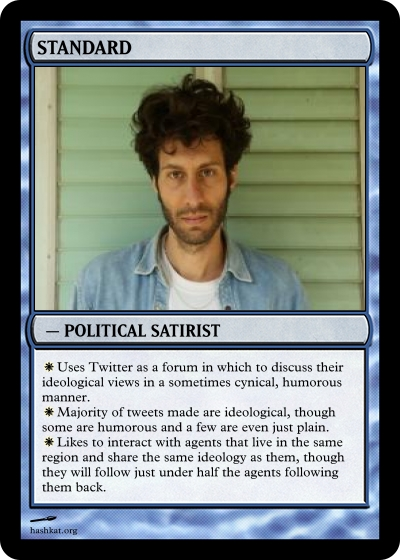``````- name: Standard-Political
weights:
# Weight with which this agent is created
# Weight with which this agent is followed in agent follow
follow: 5.0
tweet_type:
ideological: 8.0
plain: 1.0
musical: 0.0
humorous: 3.0
# Probability that following this agent results in a follow-back
followback_probability: 0.44
hashtag_follow_options:
care_about_ideology: true # does the agent care about the ideology of the agent they will follow?
rates:
# Rate for follows from this agent:
follow: {function: constant, value: 0.01}
# Rate for tweets from this agent:
tweet: {function: constant, value: 0.12}
``````

Make sure that your tweet, retweet, and follow ranks have also been updated to properly account for this network.

As you've probably noticed, we've set the max_real_time to 10 minutes instead of the usual 1 minute. The simulation should not run for this long, we just want to allow enough time for the simulation to run to completion. When running this simulation on our computer it took approximately 4 minutes for the simulated time to elapse. It is not necessary for simulated time to elapse when running a simulation. You may change the max_real_time to a time of your choosing or press Ctrl-c at any time during a simulation to stop it.

Once you have finished configuring INFILE.yaml, run and visualize your network simulation.

After running the network simulation to completion, we produced the following network visualization: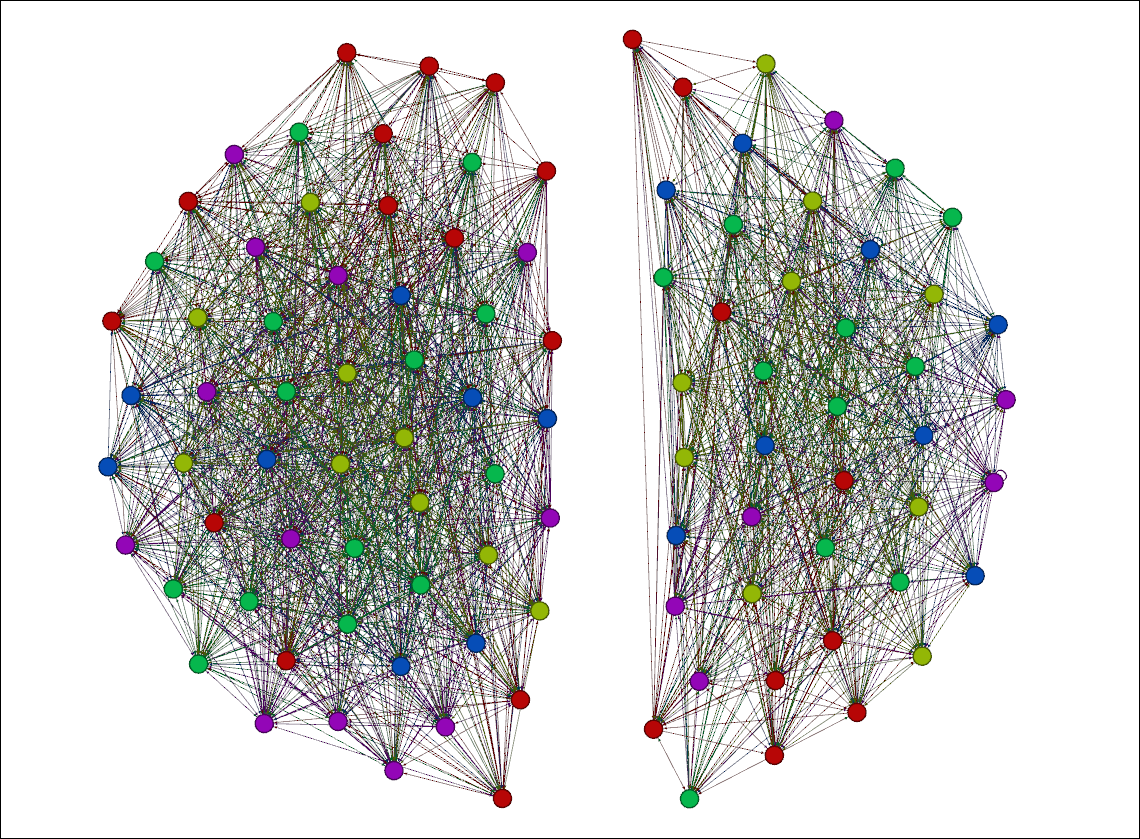In the above visualization, the red nodes correspond to the 'Standard-Chatty' agents, the green nodes correspond to the 'Standard-Friendly' agents, the yellow nodes correspond to the 'Standard-Funny' agents, the blue nodes correspond to 'Standard-Musical' agents, and the purple nodes correspond to the 'Standard-Political' agents.

The social network is two sub-networks with no intersection. This reflects the two languages and lack of bilingual agents.

By clicking the 'Filters' tab on the right-hand side of the page in Gephi and clicking 'Degree-Range' under 'Topology', we can filter our network visualization based on the cumulative-degree of the agents in the network.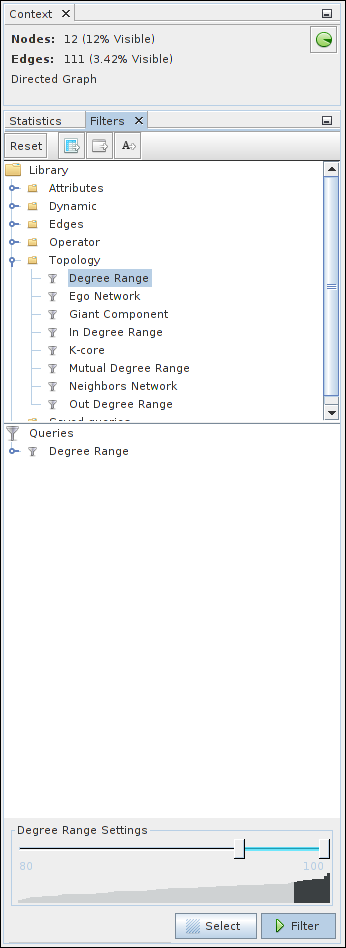Setting the 'Degree-Range Settings' from 80 to 100 and clicking 'Filter', we can see the following: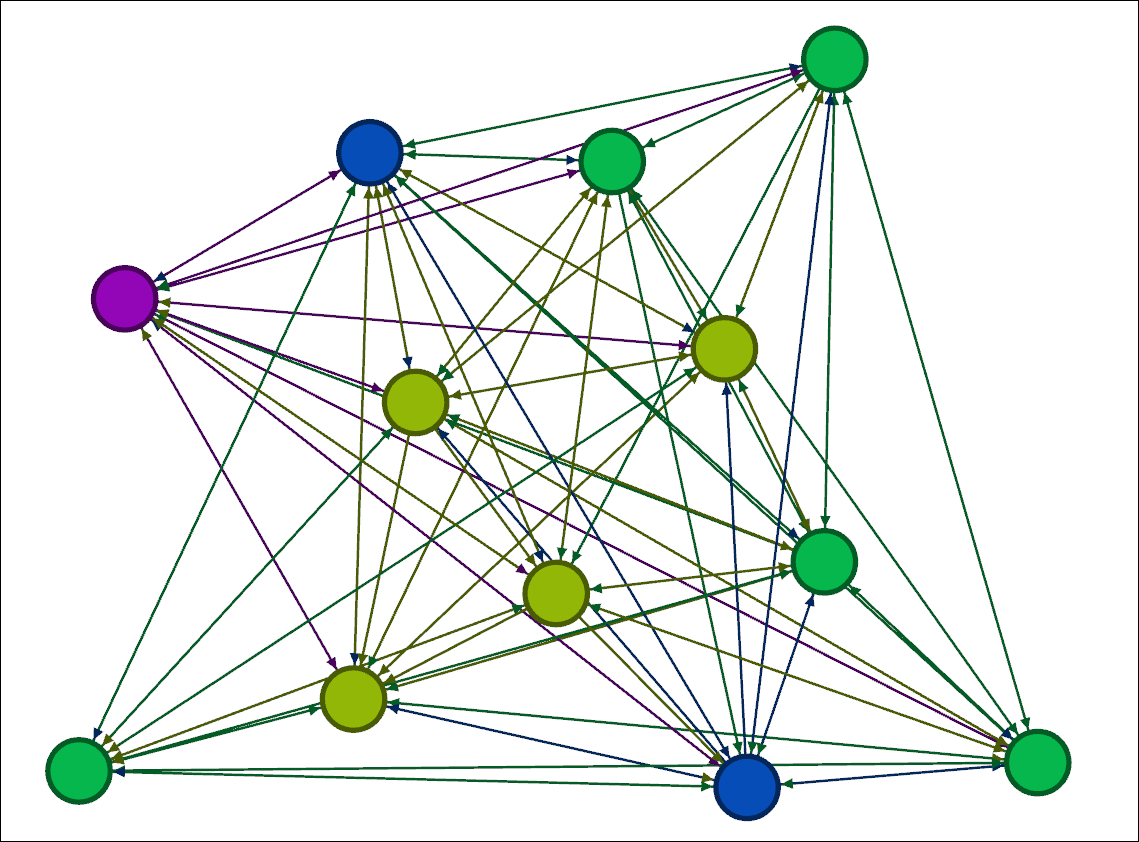As we can see from this visualization that the most highly connected nodes are the green and yellow nodes, the 'Standard-Friendly' and 'Standard-Funny' agents.

Looking into the region_connection_matrix_month_000.dat output file, we can see the connections agents made within and between regions: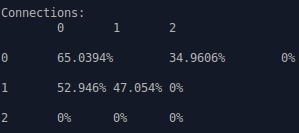As we can see, agents in Ontario, the zeroth region, had 65.0394% of their follows from agents from Ontario and 34.9609% from agents in Quebec, while agents in Quebec had 52.946% of their follows from agents in Ontario and 47.054% from agents in Quebec. Ontario had a higher population of re-tweeters, and Ontarians may have gained more followers this way.

#### Next Steps

With the completion of this tutorial, you now have knowledge and experience with agent configuration. In the next tutorial we demonstrate more complex situations that may be modeled in #k@.